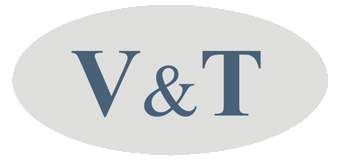Leader of drives, control and power solution, as ABB, Siemens, Control Techniques, Danfoss
 Home  |About  |Products  |Application  |Clients & References  |News  |Support  |FAQ  |Contact

## Application of V&T EcoDriveCN® variable speed drives for CNC engraving machines

Photos on site of open loop or closed loop control application cases of machine tools

Site conditions

The machinery: CNC engraving machine

V&T EcoDriveCN® vector control variable speed drives: V5-H-4T3.7G/5.5L-H3 (Please kindly notice that this is a special example. No power supply of 200V is available there...)

Motor nameplate: 200V, 133Hz, 2000r/min, 5.6A

Wiring diagram of our drive on this engraving machine
...

System solution

Adopt vector control 1 to control engraving machine. The line speed is given by AI1 analog terminal. The start/stop is controlled by digital terminal X1. Corectly set the motor parameters of variable speed drive, controlled by multi-steps V/F curve. Thus the engraving machine can get stronger torque.

Through the motor nameplate, we can calculate the base running frequency of motor, and the group of P9 motor parameters: The base running frequency of motor f: 133/200=X/380. So X = 252.7Hz. We set f = 250Hz.

Motor poles: n = 60*f/n = 60*133/2000 = 3.99. So the number of pole pairs of motor is 4. So this is 8 pole motor. P9.01 = 8. Rated speed of motor: r1 = 60*250/4 = 3750r/min. Because there's error for the real rated speed, generally smaller, the real rated speed can be set, r2 = 3700r/min. That is, P9.02 = 3700 r/min.

...

Function code setting

The original function code parameters are: P0.03=0, P0.04=1, P0.06=1, P0.08=5, P0.09=5, P0.11=400, P0.13=400, P0.14=5, P0.15=400HZ, P5.00=2, P9.01=4, P9.02=1500 r/min, P9.03=3.7 kW, P9.04=7.7A, P9.05=4.0A

According to this setting, the torque of grinding castings is not strong. We notice the running parameters of V&T variable speed drive: running frequency is 106.8Hz, the output current is varied in the range of 0.9A ~ 1.1A.

The correct function parameters are: ...

If set a V/F multi-step curve point, can increase the torque of variable speed drive better: P4.00=1, P4.05=133HZ, P4.06=58%, P4.07=250HZ, P4.08=100%. Now the running parameters of V&T variable speed drive: running frequency is 80Hz, the output current is varied from 1.8A to 2.0A. The torque of grinding castings is increased obviously.

The V/F curve boost diagram:
...

Attention

From the above example of different parameters setting of variable speed drive, it's important for input the correct parameters for the drive according to motor nameplate. This makes the torque of motor larger, thus meeting the demands of the customers better.

The parameters setting of V/F curve is better if the according voltage of the frequency is increased 10%. For example, 133Hz is for 200V. Then increase the voltage to 200V, this will be better. ...

During the adjustment, note the output current and running frequency of variable speed drive, and observe the surface of grinding castings. This is the factors to evaluate if the parameters setting can meet the requirement.

Some information is not presented on the web page here. For details, just download this document: Application of V&T EcoDriveCN® drives for engraving machine.

Our varispeed drives are applied in the machine tool industry in the world, including cold forging pressess, rolling machines, lathe, drilling machine, CNC drilling machine, boring machine, cable making tool, chamfer machine, engraving machine, grinding machine, milling machine, shearing machine, die casting machine, drawing machine, high-speed drilling machine, multi-spindle drilling machine, electric discharge machine, etching machines, gear cutting machines, lapping machines, milling machines, planing machines, etc.Photo Gallery  |CE certificate  |Superb components